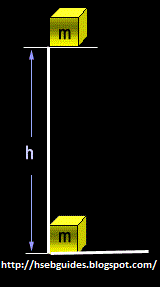## TyroCity

Physics XI Notes for Physics Notes

Posted on • Updated on

# Energy

“The ability of a body to perform work is called Energy”.
A body cannot perform work if it does not posses energy. A body cannot perform work more than the amount of energy.
It is a scalar quantity.

Units of energy

1. Joule
2. Calorie [NOTE: 1 Calorie = 4.2 joule.]
3. KWatt-Hour

Types of energy
There are numerous types of energy such as:

• Heat Energy
• Light Energy
• Sound Energy
• Nuclear Energy
• Chemical Energy
• Electrical Energy
• Solar Energy
• Wind Energy
• Kinetic Energy
• Potential Energy etc.

Power
“The rate of work done of a body is called Power”.

Average power
Average power of a body doing work is numerically equal to the total work done divided by the time taken to perform the work.
Mathematically
Power = Work done/time
Power = Work/t
but [work = Fd]
therefore
Power = Fd/t

Units of power

1. Watt [1 watt = 1joule/sec ]
2. Kilo watt [1Kw = 1000 watt]
3. Mega watt (Mw) [1Mw = 106 watt]
4. Horse power [1Hp = 746w]

Potential energy
Introduction
Energy stored by a body by any means is called “Potential Energy”.

Definition
“The energy stored by a body due to its position in gravitational field is known as ‘Gravitational Potential Energy’”.

Formula
Consider a body of mass “m” placed at a height of “h” from the surface of earth.
Force = Weight = W
but displacement (d) = hWork done = Fd
OR
Work done = Wh
[but W = mg]
work done = mgh
We know that the work done in lifting the body is stored in the body in the form of Potential Energy. Thus, P.E. = mgh